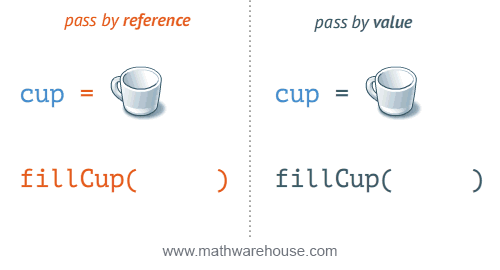# Local/global variable behaviour of lists

(This might be, in some way, related to this bug).

I recently discovered the following behaviour, which I found quite surprising - if this is intentional, can someone please explain to me why this is supposed to happen?

def bla(x):
x = 2

def bla2(K):
K = 2

def bla3(K):
T = K
T = 2


Defining

x = 1
L = 


and then running bla(x) does not change the value of x, but running bla2(L) or bla3(L) DOES change the value of L to .

edit retag close merge delete

Sort by » oldest newest most voted

Hello, @Bob67846! This is not a bug at all. This is the normal behavior of Python that is inherited by Sage. In Python, arguments are passed by value. Let me explain. This is a quite technical explanation, so I hope not to confuse you.

In order to understand better, let me alter your definition of bla a little bit:

x = 1
def bla(y):
y = 2
print(x)


When you call something like bla(x), you are passing x by value, meaning that the value of the variable x (which is 1) is "copied into" the variable y (in formal terms, you are creating an object called y, which references the value 1). Then, you reassign y to reference an object with the value 2, but you haven't changed the actual value of x.

Now, with that in mind, let's define bla as you did:

x = 1
def bla(x):
x = 2
print(x)


In this case, the value of x (which is 1) is copied to a new variable coincidentally called x, too, but which is a totally different object from your external x. The "internal" x is then assigned the value 2, but that doesn't alter your other x. When the function bla ends, the internal x is out of scope, and the external one is back to scope.

This is a quite different behavior from some languages, like C++, Fortran, etc., that allow you to pass by reference. If that were the case, the variable x would change its value to 2. Indeed, if bla passed arguments by reference, then y would be not a copy of the value 1, but a reference to the address in computer memory where the 1 is store. So, when you say y=2, you are saying "change the value stored in that address". In that case, x, which is still pointing to the same address, would show 2 as its value, too. This explanation is also valid with the internal/external x variables.

Now, why does bla2 actually changes the value of a list like L? The thing about lists in Python is that their values are a reference to the memory where they are stored. That is, when you execute

x = 1
L = [1, 2, 3]


the name x references the value 1, but the name L references the address in memory where the list [1, 2, 3] is created. When you call bla2(L), as with any function call in Python, you are passing the value of L (a memory address) to the variable K. As your bla2 modifies K, then it is storing the new value in the same memory address, which is also referenced by L. So L also changes.

In computational jargon, x = 1 is immutable and L = [1, 2, 3] is mutable. And knowing which types of objects are mutable or immutable is very important while programming.

The following image explains better the pass by reference vs. pass by value problem. (I always knew I would find it a use!)more

1

You could alter the value of an immutable variable by reassigning it. For example:

x = 1
def bla(x):
x = 2
return x
x = bla(x)
print(x)


This will change x to 2. However, doing the same for a mutable variable is like doing nothig:

L = [1, 2, 3]
def blabla(K):
K = -3
return K
L = blabla(L)
print(L)


works exactly as

L = [1, 2, 3]
def bla2(K):
K = -3
print(L)


This is clear, thanks!

This leaves me with two minor questions: . Do you know why variables are immutable and lists are mutable by design? At first glance it might seem more useful (?) if the changes to a list remain "local" to the function, just like the changes to the variable x in bla . What are elegant ways to write functions like bla2 without modifying L? Tuples don't seem to work for this purpose, so instead of bla3 just copy L inside the function via K = L[:]?

1

Hello, @Bob67846! Why lists are mutable? That's a good question. Just now, I realize I never made myself that question. I can think of one reason: Lists are intended to mimic arrays (in the sense of C or Java, for example). The most useful operations on arrays are appending, indexing/slicing, popping, etc. All of those imply mutability as requisite. Another reason I can think of is that arrays tend to be really large on memory, so having to copy them (i.e., making them immutable) is not only slow, but also memory consuming. Every time you would want to change an entry of the list would require you to create a copy of the list, thus doubling the memory space consumed. Also the time required to copy a list is proportional to its length!

1

If you want to create a function that works with the entries of the list L, you have to copy L by value. One way as you suggest: K = L[:]; the other way is to say K = L.copy(). But remember, this is time-and-space-consuming.

Unfortunately, immutable objects don't support item assingments like K=-3, so you have to make a copy, if you don't want to change it.